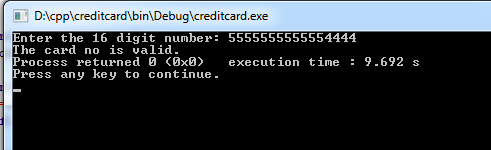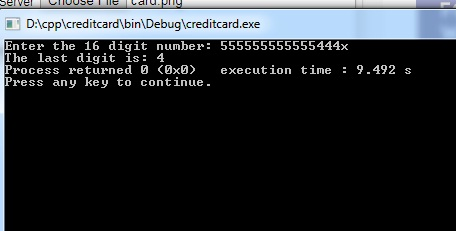This program checks the validity of a 16-digit credit card number, using the Luhn algorithm. It also calculates the final (checksum) digit, if given only the first 15 digits.

Don't use plagiarized sources. Get Your Custom Essay on
GET AN ESSAY WRITTEN FOR YOU FROM AS LOW AS \$13/PAGE

The learning objectives of the program are:

Write a complete C program.

Use loops and conditional statements.

Use arithmetic operators.

Use printf and getchar to perform I/O.

This program will work in two ways. First, if the user enters a 16-digit credit card number, the program will say whether the number is valid or not. Second, if the user enters only a 15-digit number followed by ‘x’, the program will calculate the final number, the check digit. All spaces are ignored.

The check digit is calculated using the Luhn algorithm, patented in 1960. One description of the algorithm:

Starting from the rightmost digit (the check digit), double every second digit. If doubling the digit results in a value greater than 9, then subtract 9 from the result. (Example, doubling 7 yields 14, and 14-9 = 5. So the result of “doubling” 7 would be 5.)

Sum all the digits (including the ones that were not doubled).

If the result modulo 10 is zero (i.e., if the result is a multiple of 10), then the number is valid.

Instead of checking the full number, suppose you want to generate the check digit. Here’s how:

Using the same method as above, sum the doubled and non-doubled digits. (NOTE: Must still count the check digit as the “first” digit, so that you double the same digits as before.)

Extract the one’s digit from the result, and subtract that value from 10.

Example: Same number as before, but now we calculate the check digit x: 4792308x

Sum = 8 + 7 + 9 + 2 + 6 + 0 + 7 = 39

One’s digit is 9. 10-9 = 1

MAKE SURE YOU USE GETCHAR() AND NOT SCANF()

card.cpp

#include <stdio.h>
#include <stdlib.h>

int main()
{
int no;
char digi;
int sum_odd=0,sum_even=0,last_digit=0;
printf(“Enter the 16 digit number: “);
//get 16 digits from user
for(int i=0;i<16;i++)
digi[i]=getchar() ;
// convert char into integers
for(int i=0;i<16;i++)
no[i]=digi[i] %48;

//check if last digit is x or not

if(!(digi==’x’)){ //if last digit is not x, then check for card number is valid or invalid

for(int i=15;i>0;i=i-2)
sum_odd+=no[i];

for(int i=14;i>=0;i=i-2){
d=no[i]*2;
if(d>9)
d=d-9;
sum_even+=d;
}

printf(“The card no is valid.”);
else
printf(“The card no is Invalid.”);
}
//if last digit is ‘x’ , then find the last digit
else
{
for(int i=13;i>0;i=i-2)
sum_odd+=no[i]; for(int i=14;i>=0;i=i-2){
d=no[iEnter the 16 digit number: 1234567891234567
The card no is Invalid.
Process returned 0 (0x0) execution time : 7.298 s
Press any key to continue.

output 2:

Enter the 16 digit number: 5555555555554444
The card no is valid.
Process returned 0 (0x0) execution time : 7.366 s
Press any key to continue.

output3:

Enter the 16 digit number: 555555555555444x
The last digit is: 4
Process returned 0 (0x0) execution time : 9.492 s
Press any key to continue.